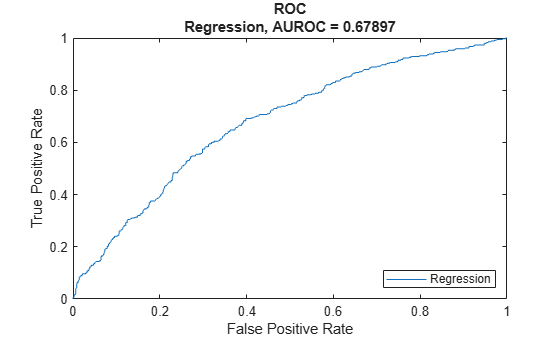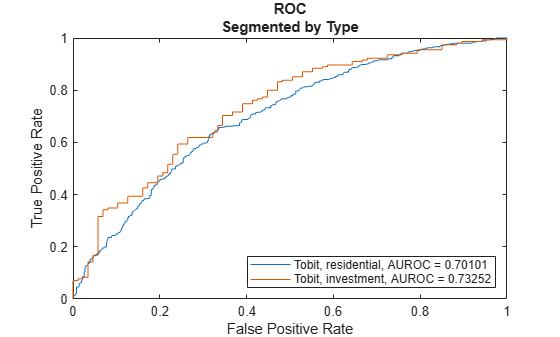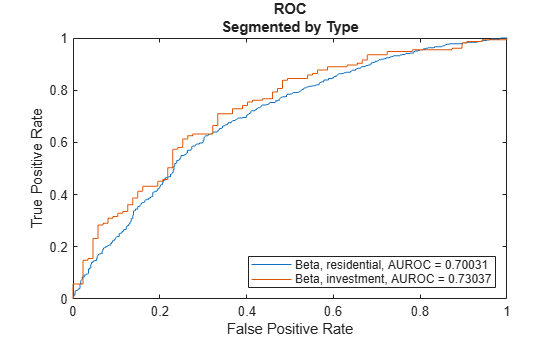# modelDiscriminationPlot

Plot ROC curve

## Syntax

``modelDiscriminationPlot(lgdModel,data)``
``modelDiscriminationPlot(___,Name,Value)``
``h = modelDiscriminationPlot(ax,___,Name,Value)``

## Description

example

````modelDiscriminationPlot(lgdModel,data)` generates the receiver operating characteristic (ROC) curve. `modelDiscriminationPlot` supports segmentation and comparison against a reference model.```

example

````modelDiscriminationPlot(___,Name,Value)` specifies options using one or more name-value pair arguments in addition to the input arguments in the previous syntax.```

example

````h = modelDiscriminationPlot(ax,___,Name,Value)` specifies options using one or more name-value pair arguments in addition to the input arguments in the previous syntax and returns the figure handle `h`.```

## Examples

collapse all

This example shows how to use `fitLGDModel` to fit data with a `Regression` model and then use `modelDiscriminationPlot` to plot the ROC.

Load the loss given default data.

```load LGDData.mat head(data)```
``` LTV Age Type LGD _______ _______ ___________ _________ 0.89101 0.39716 residential 0.032659 0.70176 2.0939 residential 0.43564 0.72078 2.7948 residential 0.0064766 0.37013 1.237 residential 0.007947 0.36492 2.5818 residential 0 0.796 1.5957 residential 0.14572 0.60203 1.1599 residential 0.025688 0.92005 0.50253 investment 0.063182 ```

Partition Data

Separate the data into training and test partitions.

```rng('default'); % for reproducibility NumObs = height(data); c = cvpartition(NumObs,'HoldOut',0.4); TrainingInd = training(c); TestInd = test(c);```

Create a `Regression` LGD Model

Use `fitLGDModel` to create a `Regression` model using training data.

```lgdModel = fitLGDModel(data(TrainingInd,:),'regression'); disp(lgdModel) ```
``` Regression with properties: ResponseTransform: "logit" BoundaryTolerance: 1.0000e-05 ModelID: "Regression" Description: "" UnderlyingModel: [1x1 classreg.regr.CompactLinearModel] PredictorVars: ["LTV" "Age" "Type"] ResponseVar: "LGD" ```

Display the underlying model.

`disp(lgdModel.UnderlyingModel)`
```Compact linear regression model: LGD_logit ~ 1 + LTV + Age + Type Estimated Coefficients: Estimate SE tStat pValue ________ ________ _______ __________ (Intercept) -4.7549 0.36041 -13.193 3.0997e-38 LTV 2.8565 0.41777 6.8377 1.0531e-11 Age -1.5397 0.085716 -17.963 3.3172e-67 Type_investment 1.4358 0.2475 5.8012 7.587e-09 Number of observations: 2093, Error degrees of freedom: 2089 Root Mean Squared Error: 4.24 R-squared: 0.206, Adjusted R-Squared: 0.205 F-statistic vs. constant model: 181, p-value = 2.42e-104 ```

Plot ROC Data

Use `modelDiscriminationPlot` to plot the ROC for the test data set.

`modelDiscriminationPlot(lgdModel,data(TestInd,:))`This example shows how to use `fitLGDModel` to fit data with a `Tobit` model and then use `modelDiscriminationPlot` to plot the ROC.

Load the loss given default data.

```load LGDData.mat head(data)```
``` LTV Age Type LGD _______ _______ ___________ _________ 0.89101 0.39716 residential 0.032659 0.70176 2.0939 residential 0.43564 0.72078 2.7948 residential 0.0064766 0.37013 1.237 residential 0.007947 0.36492 2.5818 residential 0 0.796 1.5957 residential 0.14572 0.60203 1.1599 residential 0.025688 0.92005 0.50253 investment 0.063182 ```

Partition Data

Separate the data into training and test partitions.

```rng('default'); % for reproducibility NumObs = height(data); c = cvpartition(NumObs,'HoldOut',0.4); TrainingInd = training(c); TestInd = test(c);```

Create a Tobit LGD Model

Use `fitLGDModel` to create a `Tobit` model using training data.

```lgdModel = fitLGDModel(data(TrainingInd,:),'tobit'); disp(lgdModel) ```
``` Tobit with properties: CensoringSide: "both" LeftLimit: 0 RightLimit: 1 ModelID: "Tobit" Description: "" UnderlyingModel: [1x1 risk.internal.credit.TobitModel] PredictorVars: ["LTV" "Age" "Type"] ResponseVar: "LGD" ```

Display the underlying model.

`disp(lgdModel.UnderlyingModel)`
```Tobit regression model: LGD = max(0,min(Y*,1)) Y* ~ 1 + LTV + Age + Type Estimated coefficients: Estimate SE tStat pValue _________ _________ _______ __________ (Intercept) 0.058257 0.027279 2.1356 0.032828 LTV 0.20126 0.031383 6.4129 1.7592e-10 Age -0.095407 0.0072435 -13.171 0 Type_investment 0.10208 0.018054 5.6544 1.7785e-08 (Sigma) 0.29288 0.0057071 51.318 0 Number of observations: 2093 Number of left-censored observations: 547 Number of uncensored observations: 1521 Number of right-censored observations: 25 Log-likelihood: -698.383 ```

Plot ROC Data

Use `modelDiscriminationPlot` to plot the ROC for the test data set.

`modelDiscriminationPlot(lgdModel,data(TestInd,:),"SegmentBy","Type","DiscretizeBy","median")`This example shows how to use `fitLGDModel` to fit data with a `Beta` model and then use `modelDiscriminationPlot` to plot the ROC.

Load the loss given default data.

```load LGDData.mat head(data)```
``` LTV Age Type LGD _______ _______ ___________ _________ 0.89101 0.39716 residential 0.032659 0.70176 2.0939 residential 0.43564 0.72078 2.7948 residential 0.0064766 0.37013 1.237 residential 0.007947 0.36492 2.5818 residential 0 0.796 1.5957 residential 0.14572 0.60203 1.1599 residential 0.025688 0.92005 0.50253 investment 0.063182 ```

Partition Data

Separate the data into training and test partitions.

```rng('default'); % for reproducibility NumObs = height(data); c = cvpartition(NumObs,'HoldOut',0.4); TrainingInd = training(c); TestInd = test(c);```

Create a `Beta` LGD Model

Use `fitLGDModel` to create a `Beta` model using training data.

```lgdModel = fitLGDModel(data(TrainingInd,:),'Beta'); disp(lgdModel) ```
``` Beta with properties: BoundaryTolerance: 1.0000e-05 ModelID: "Beta" Description: "" UnderlyingModel: [1x1 risk.internal.credit.BetaModel] PredictorVars: ["LTV" "Age" "Type"] ResponseVar: "LGD" ```

Display the underlying model.

`disp(lgdModel.UnderlyingModel)`
```Beta regression model: logit(LGD) ~ 1_mu + LTV_mu + Age_mu + Type_mu log(LGD) ~ 1_phi + LTV_phi + Age_phi + Type_phi Estimated coefficients: Estimate SE tStat pValue ________ ________ _______ __________ (Intercept)_mu -1.3772 0.13201 -10.433 0 LTV_mu 0.60269 0.15087 3.9947 6.7023e-05 Age_mu -0.47464 0.040264 -11.788 0 Type_investment_mu 0.45372 0.085143 5.3289 1.094e-07 (Intercept)_phi -0.16337 0.12591 -1.2975 0.19462 LTV_phi 0.055892 0.14719 0.37973 0.70419 Age_phi 0.22887 0.040335 5.6743 1.5863e-08 Type_investment_phi -0.14102 0.078155 -1.8044 0.071311 Number of observations: 2093 Log-likelihood: -5291.04 ```

Plot ROC Data

Use `modelDiscriminationPlot` to plot the ROC for the test data set.

`modelDiscriminationPlot(lgdModel,data(TestInd,:),"SegmentBy","Type","DiscretizeBy","median")`## Input Arguments

collapse all

Loss given default model, specified as a previously created `Regression`, `Tobit`, or `Beta` object using `fitLGDModel`.

Data Types: `object`

Data, specified as a `NumRows`-by-`NumCols` table with predictor and response values. The variable names and data types must be consistent with the underlying model.

Data Types: `table`

(Optional) Valid axis object, specified as an `ax` object that is created using `axes`. The plot will be created in the axes specified by the optional `ax` argument instead of in the current axes (gca). The optional argument `ax` must precede any of the input argument combinations.

Data Types: `object`

### Name-Value Arguments

Specify optional pairs of arguments as `Name1=Value1,...,NameN=ValueN`, where `Name` is the argument name and `Value` is the corresponding value. Name-value arguments must appear after other arguments, but the order of the pairs does not matter.

Before R2021a, use commas to separate each name and value, and enclose `Name` in quotes.

Example: `modelDiscriminationPlot(lgdModel,data(TestInd,:),'DataID','Testing','DiscretizeBy','median')`

Data set identifier, specified as the comma-separated pair consisting of `'DataID'` and a character vector or string. The `DataID` is included in the output for reporting purposes.

Data Types: `char` | `string`

Discretization method for LGD `data`, specified as the comma-separated pair consisting of `'DiscretizeBy'` and a character vector or string.

• `'mean'` — Discretized response is `1` if observed LGD is greater than or equal to the mean LGD, `0` otherwise.

• `'median'` — Discretized response is `1` if observed LGD is greater than or equal to the median LGD, `0` otherwise.

• `'positive'` — Discretized response is `1` if observed LGD is positive, `0` otherwise (full recovery).

• `'total'` — Discretized response is `1` if observed LGD is greater than or equal to `1` (total loss), `0` otherwise.

Data Types: `char` | `string`

Name of a column in the `data` input, not necessarily a model variable, to be used to segment the data set, specified as the comma-separated pair consisting of `'SegmentBy'` and a character vector or string. One AUROC is reported for each segment, and the corresponding ROC data for each segment is returned in the optional output.

Data Types: `char` | `string`

LGD values predicted for `data` by the reference model, specified as the comma-separated pair consisting of `'ReferenceLGD'` and a `NumRows`-by-`1` numeric vector. The ROC curve is plotted for both the `lgdModel` object and the reference model.

Data Types: `double`

Identifier for the reference model, specified as the comma-separated pair consisting of `'ReferenceID'` and a character vector or string. `'ReferenceID'` is used in the plot for reporting purposes.

Data Types: `char` | `string`

## Output Arguments

collapse all

Figure handle for the line objects, returned as handle object.

collapse all

### Model Discrimination Plot

The `modelDiscriminationPlot` function plots the receiver operator characteristic (ROC) curve.

The `modelDiscriminationPlot` function also shows the area under the receiver operator characteristic (AUROC) curve, sometimes called simply the area under the curve (AUC). This metric is between 0 and 1 and higher values indicate better discrimination.

A numeric prediction and a binary response are needed to plot the ROC and compute the AUROC. For LGD models, the predicted LGD is used directly as the prediction. However, the observed LGD must be discretized into a binary variable. By default, observed LGD values greater than or equal to the mean observed LGD are assigned a value of 1, and values below the mean are assigned a value of 0. This discretized response is interpreted as "high LGD" vs. "low LGD." The ROC curve and the AUROC curve measure how well the predicted LGD separates the "high LGD" vs. the "low LGD" observations. The discretization criterion can be changed with the `DiscretizeBy` name-value pair argument for `modelDiscriminationPlot`.

The ROC curve is a parametric curve that plots the proportion of

• High LGD cases with predicted LGD greater than or equal to a parameter t, or true positive rate (TPR)

• Low LGD cases with predicted LGD greater than or equal to the same parameter t, or false positive rate (FPR)

The parameter t sweeps through all the observed predicted LGD values for the given data. If the AUROC value or the ROC curve data are needed programmatically, use the `modelDiscrimination` function. For more information about ROC curves, see ROC Curve and Performance Metrics.

 Baesens, Bart, Daniel Roesch, and Harald Scheule. Credit Risk Analytics: Measurement Techniques, Applications, and Examples in SAS. Wiley, 2016.

 Bellini, Tiziano. IFRS 9 and CECL Credit Risk Modelling and Validation: A Practical Guide with Examples Worked in R and SAS. San Diego, CA: Elsevier, 2019.